# Ultrasonic Sensor and Temperature Compensation

If you've been using Arduino, you might have used or have heard about the ultrasonic sensor. Most of us use it for level/distance measurement while some use it to detect an obstacle in front. What most of us don’t know is that there is an underlying flaw with the ultrasonic sensor, particularly on the first application I mentioned (level/distance measurement).

The HC-SR04 ultrasonic sensor is able to detect an object in front of it by emitting an ultrasound and then waiting for that ultrasound to bounce back. Naturally, the further the object, the longer the “echo” to arrive.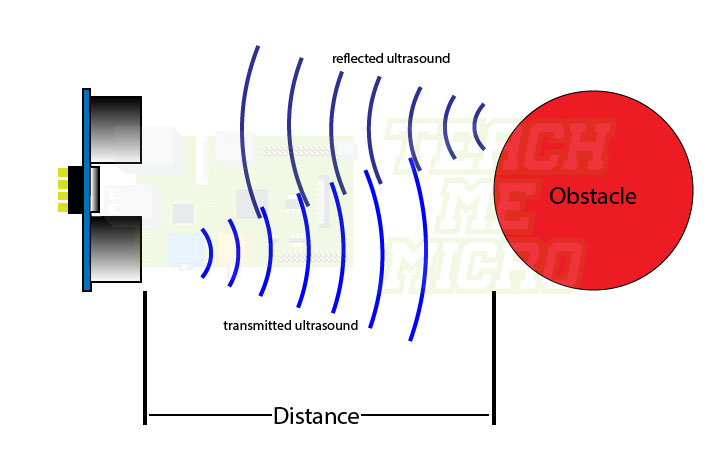Most tutorials (including my own), uses this formula to determine the distance of the obstacle:



Here, 0.034 is the speed of sound in centimeters per microsecond. So when the “echo” returns in 100 microseconds (t in the formula), the distance of the obstacle that bounced the ultrasound is 1.7 centimeters.

### Speed of Sound and Temperature

The error lies in the value of the speed of sound because this value is actually temperature dependent. In fact, the practical formula of the speed of sound is:Where T is the temperature of the surrounding in degree Celsius. Moreover, the usual speed of 0.034 cm/us or 340 m/s, using this formula, only works when the temperature is 14.35 °C!

Now let’s see the measurement difference between the two formulas. The old one, as mentioned, detects the obstacle to be at 17 centimeters. For the new formula, I will be using today’s temperature which is 33 °C (yeah it’s hot here). This gives me a speed of sound value ofConsequently, the distance of the object would be: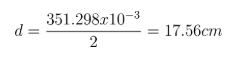A 0.56 centimeter difference! Also, this difference may vary during different times of the day as the temperature varies.

So what I did is install a temperature sensor with my ultrasonic sensor to compensate for any temperature difference. Here’s my setup: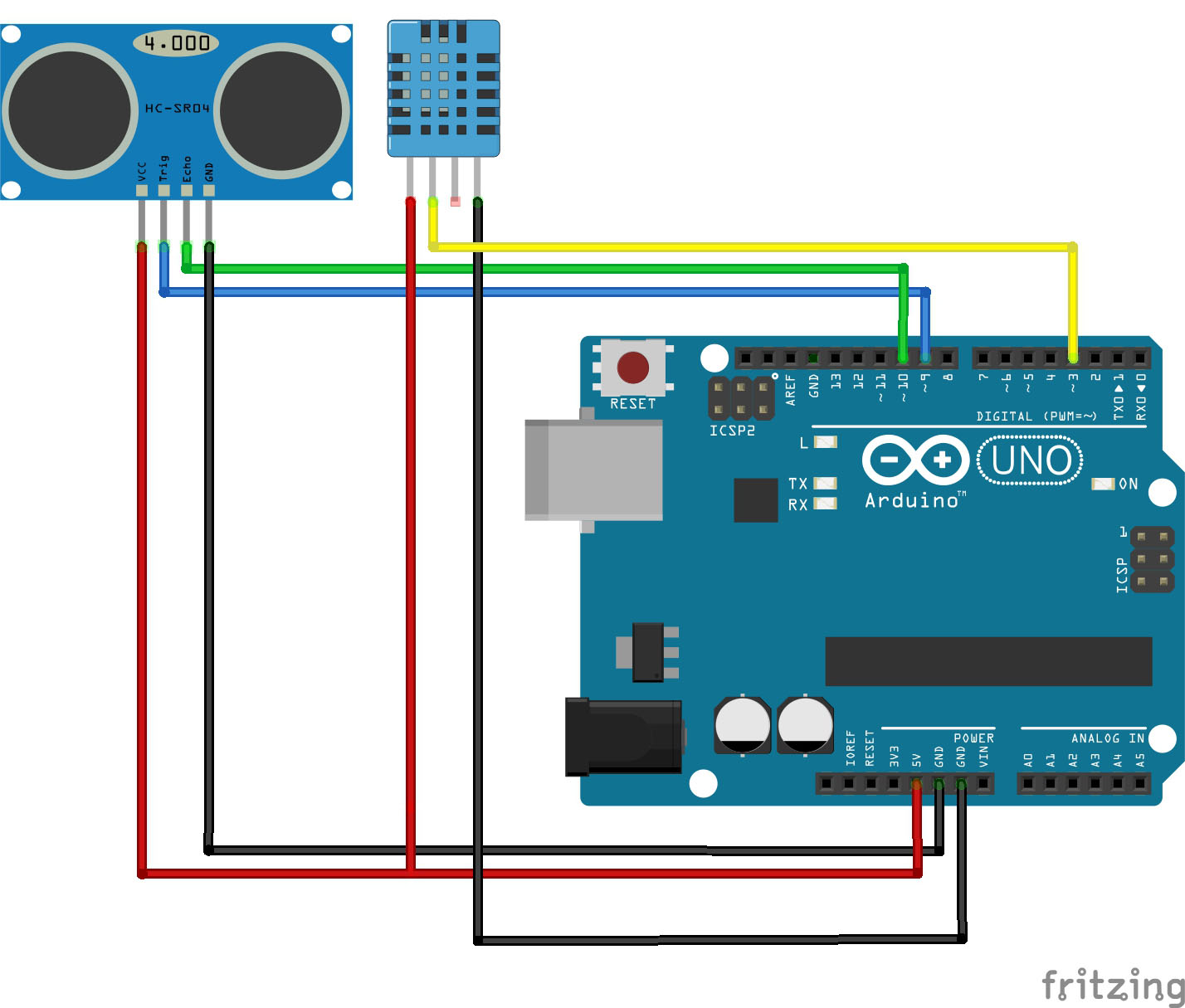I used a DHT11 to measure the ambient temperature and to compensate for the temperature effects to the ultrasonic sensor.

The sketch for this project is down below. For those looking to replicate this project, you need to download the Adafruit Unified Sensor and DHT libraries.

// Level/Obstacle Distance Measuring Using Ultrasonic Sensor and DHT11
// by R. Pelayo
// from www.teachmemicro.com
// Released under an MIT license.

// REQUIRES the following Arduino libraries:
// - DHT Sensor Library: https://github.com/adafruit/DHT-sensor-library

#include <DHT.h>
#include <DHT_U.h>

#define DHTPIN 2     // Digital pin connected to the DHT sensor
#define TRIGGER 9    // Ultrasonic sensor trigger pin
#define ECHO 10      // Ultrasonic sensor echo pin

#define DHTTYPE    DHT11     // DHT 11

DHT_Unified dht(DHTPIN, DHTTYPE);

uint32_t delayMS;
long duration;
float distance;
float speed_sound;

void setup() {
// Set pin modes
pinMode(TRIGGER, OUTPUT);
pinMode(ECHO, INPUT);
Serial.begin(9600);
// Initialize device.
dht.begin();
Serial.println(F("Distance Measuring with Temperature Compensation"));
// Declare sensor object
sensor_t sensor;
dht.temperature().getSensor(&sensor);
delayMS = sensor.min_delay / 1000;   //delay between measurements
}

void loop() {
// Delay between measurements.
delay(delayMS);
// Get temperature event and print its value.
sensors_event_t event;
dht.temperature().getEvent(&event);
//Serial.print(event.temperature);
// Clears the TRIGGER
digitalWrite(TRIGGER, LOW);
delayMicroseconds(2);
// Sets the TRIGGER on HIGH state for 10 micro seconds
digitalWrite(TRIGGER, HIGH);
delayMicroseconds(10);
digitalWrite(TRIGGER, LOW);
// Reads the ECHO , returns the sound wave travel time in microseconds
duration = pulseIn(ECHO, HIGH);
speed_sound = 331.3 + 0.606*(float)event.temperature;
speed_sound = speed_sound / 10000;  // convert to centimeters per microsecond
// Calculating the distance
distance= duration*speed_sound/2;
// Prints the distance on the Serial Monitor
Serial.print("Distance: ");
Serial.println(distance);
}

Naturally, I had to test this sketch. Here’s a picture of my actual setup: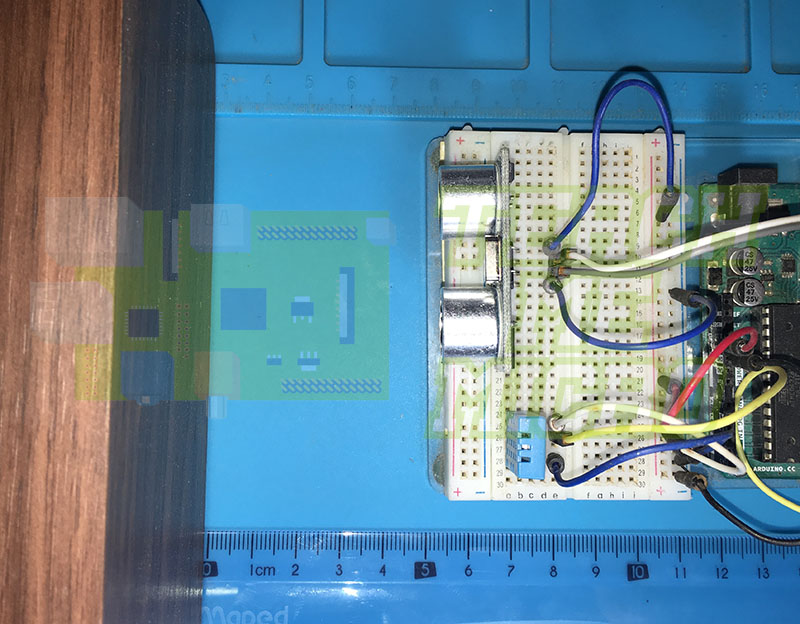The distance from the sensor to the obstacle is around 5.4 centimeters (as shown by the ruler). Here are the results if there's no temperature compensation: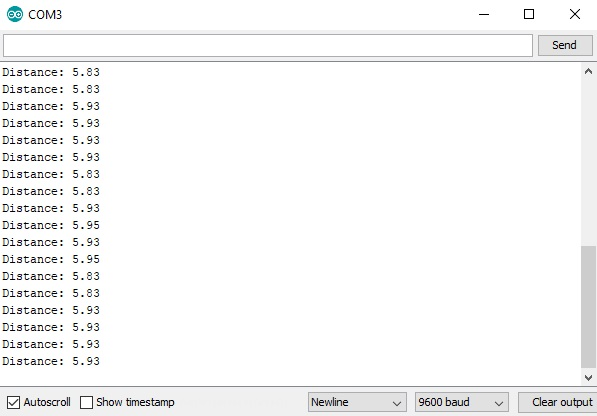The sensor reads a distance of around 5.9 centimeters, and 0.5 centimeter difference. Now here’s the results with temperature compensation:The sensor now reads a distance of around 5.4 centimeters!